# Mathematical proof facts for kids

Kids Encyclopedia Facts
(Redirected from Proof (mathematics))

A mathematical proof is a way to show that a math theorem is true. One must show that the theory is true in all cases.

There are different ways of proving a mathematical theorem.

## Proof by Induction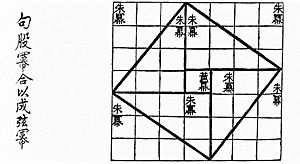Visual proof for the (3, 4, 5) triangle as in the Zhoubi Suanjing 500–200 BC.

One type of proof is called proof by induction. This is usually used to prove a theorem that is true for all numbers. There are 4 steps in a proof by induction.

1. State that the proof will be by induction, and state which variable will be used in the induction step.

2. Prove that the statement is true for some beginning case.

3. Assume that for some value n = n0 the statement is true and has all of the properties listed in the statement. This is called the induction step.

4. Show that the statement is true for the next value, n0+1.

Once that is shown, then it means that for any value of n that is picked, the next one is true. Since it's true for some beginning case (usually n=1), then it's true for the next one (n=2). And since it's true for 2, it must be true for 3. And since it's true for 3, it must be true for 4, etc. Induction shows that it is always true, precisely because it's true for whatever comes after any given number.

An example of proof by induction:

Prove that for all natural numbers n, 2(1+2+3+....+n-1+n)=n(n+1)

Proof: First, the statement can be written "for all natural numbers n, 2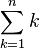$\sum_{k=1}^n k$=n(n+1)

By induction on n,

First, for n=1, 2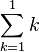$\sum_{k=1}^1 k$=2(1)=1(1+1), so this is true.

Next, assume that for some n=n0 the statement is true. That is, 2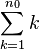$\sum_{k=1}^{n_0} k$ = n0(n0+1)

Then for n=n0+1, 2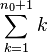$\sum_{k=1}^{{n_0}+1} k$ can be rewritten 2(n0+1) + 2$\sum_{k=1}^{n_0} k$

Since 2$\sum_{k=1}^{n_0} k$ = n0(n0+1), 2n0+1 + 2$\sum_{k=1}^{n_0} k$ = 2(n0+1) + 2n0(n0+1)

So 2(n0+1) + 2n0(n0+1)= 2(n0+1)(n0 + 2)

Proof by contradiction is a way of proving a mathematical theorem by showing that if the statement is false, there is a problem with the logic of the proof. That is, if one of the results of the theorem is assumed to be false, then the proof does not work.

When proving a theorem by way of contradiction, it is important to note that in the beginning of the proof. This is usually abbreviated BWOC. When the contradiction appears in the proof, there is usually an X made with 4 lines instead of 2 placed next to that line.

## Other Examples of Proofs

Prove that x = -b ± ( √b² - 4ac ) / 2a from ax²+bx+c=0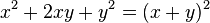$x^2+2xy+y^2 = (x+y)^2\!$.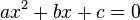$ax^2+bx+c=0\!$

by a (which is allowed because a is non-zero), gives: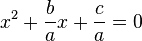$x^2 + \frac{b}{a} x + \frac{c}{a}=0\!$

or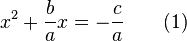$x^2 + \frac{b}{a} x= -\frac{c}{a} \qquad (1)\!$

The quadratic equation is now in a form in which completing the square can be done. To "complete the square" is to find some number k so that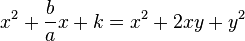$x^2 + \frac{b}{a}x + k = x^2+2xy+y^2\!$

for another number y. In order for these equations to be true,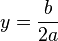$y = \frac{b}{2a}\!$

and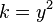$k = y^2\!$

so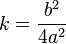$k = \frac{b^2}{4a^2}\!$.

Adding this number to equation (1) makes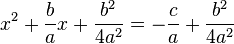$x^2+\frac{b}{a}x+\frac{b^2}{4a^2}=-\frac{c}{a}+\frac{b^2}{4a^2}\!$.

The left side is now a perfect square because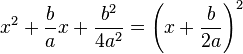$x^2+\frac{b}{a}x+\frac{b^2}{4a^2} = \left( x + \frac{b}{2a} \right)^2\!$

The right side can be written as a single fraction, with common denominator 4a2. This gives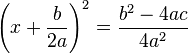$\left(x+\frac{b}{2a}\right)^2=\frac{b^2-4ac}{4a^2}\!$.

Taking the square root of both sides gives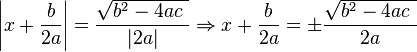$\left|x+\frac{b}{2a}\right| = \frac{\sqrt{b^2-4ac\ }}{|2a|}\Rightarrow x+\frac{b}{2a}=\pm\frac{\sqrt{b^2-4ac\ }}{2a}\!$.

Getting x by itself gives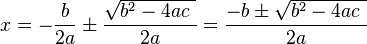$x=-\frac{b}{2a}\pm\frac{\sqrt{b^2-4ac\ }}{2a}=\frac{-b\pm\sqrt{b^2-4ac\ }}{2a}\!$.

## Images for kidsMathematical proof Facts for Kids. Kiddle Encyclopedia.